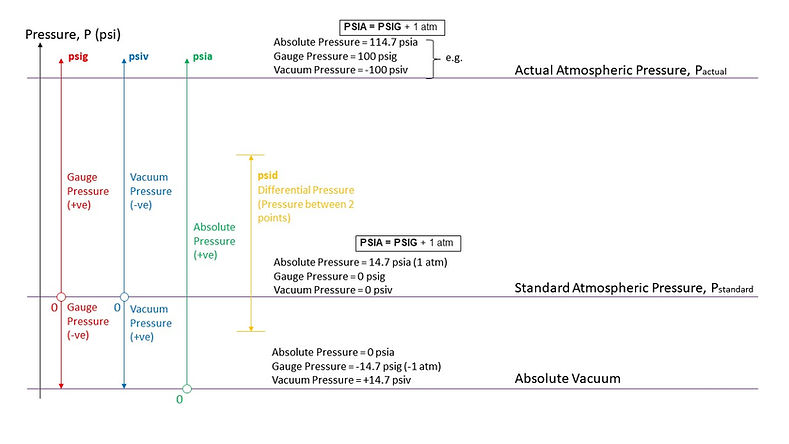top of pageStandard CFM to Actual CFM Converter

Actual Cubic Feet per Minute (ACFM) is the volumetric flowrate of a gas that is independent on density.

At standard conditions, i.e. standard temperature and pressure, here set at Pstd = 20 ºC (68 ºF), 14.7 psia (101.325 kPa), 0% RH, Actual CFM (ACFM) is equal to Standard CFM (SCFM).

ACFM = SCFM Standard PressureIn actual fact, when a space has more positive pressure (higher than Pstd (101,325 Pa), ACFM is lower than its SCFM. This is because when a space has more positive pressure, the air is more compressed. This means that the same mass of air occupies lower volume of air. In other words, more positive pressure air has higher density.

ACFM < SCFM (+ve Pressure)

On the other hand, when a space is more negative or closer resembles a vacuum, ACFM is higher than its SCFM. This is because the volume of air for the same mass of gas increases, or in other words the density of air increases.

ACFM > SCFM ( -ve Pressure)

Therefore, as mentioned, the actual CFM (ACFM) considers the "actual" volume of the gas independent of the gas density.

The relationship between Actual CFM (ACFM) and Standard CFM (SCFM) can be represented by the equation shown below. Actual CFM corresponds to “Actual Condition” stated below, while Standard CFM corresponds to “Standard Conditions”, which are set at 68ºF, 14.696 psia and 0% RH.All units of Pressure used in this equation are Absolute Pressure in pounds per inch squared (Absolute) (psia). Note that there are difference between absolute pressure (psia), gauge pressure (psig) (or barometric pressure) and vacuum pressure (psiv).

Pactual can be related to the elevation altitude (meter), i.e. the height above sea level where the measurement of the actual pressure is taken, through this link.PSIA is the absolute pressure measured from zero point at absolute vacuum, i.e. 0 psia, or -14.7 psig

PSIG is the gauge pressure measured up positively from zero point at standard atmospheric pressure, Pstandard, i.e. 1 atm or 14.7 psia or 0 psig.

PSIV is the vacuum pressure measured down positively from zero point at standard atmospheric pressure, Pstandard, i.e. 1 atm or 14.7 psia or 0 psig.

PSID is the differential pressure between any 2 points of pressure level. Unit's in psi.bottom of page